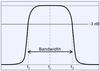RLC振荡回路

band-pass filter

• ## 带通滤波器的设计

万次阅读 2019-08-09 12:17:18
一、滤波器：滤波器按照频带划分可以分为：低通滤波器(LPF)、高通滤波器(HPF)、带通滤波器(BPF)、带阻滤波器(BEF)。其中射频天线领域主要采用带通滤波器(BPF)。 二、带通滤波器(BPF)： ①：RF BPF：从天线中...
一、滤波器：滤波器按照频带划分可以分为：低通滤波器(LPF)、高通滤波器(HPF)、带通滤波器(BPF)、带阻滤波器(BEF)。其中射频天线领域主要采用带通滤波器(BPF)。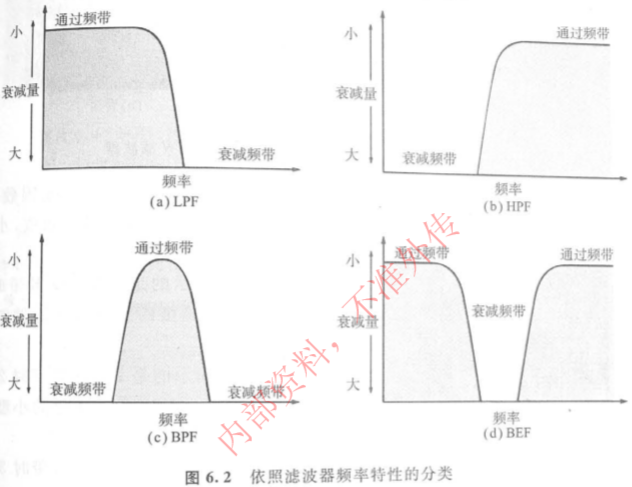二、带通滤波器(BPF)：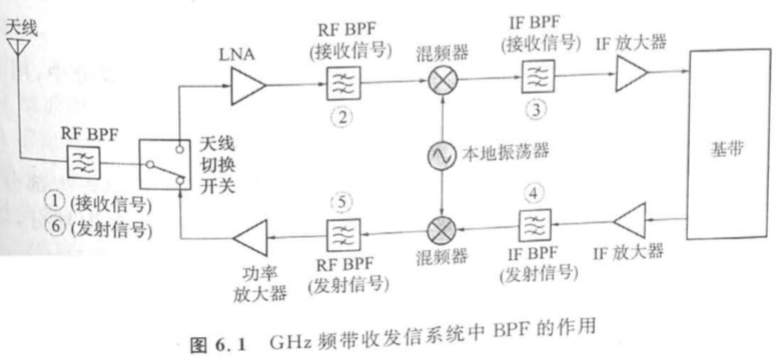①：RF BPF：从天线中取出期望频带的有效信号，滤除不需要频带的电磁波信号和噪声。

②：RF BPF：用下级混频器(降频混频器)进行频率转换时，防止在IF频带中引入干扰信号，用BPF将不期望频率成分滤除。

③：IF BPF：从混频器进行频率转换所产生的许多频率成分中，用BPF只取出期望的IF信号，也具有限制接收信号频带的作用。

④：IF BPF：用混频器(升频转换器)进行频率转换时，用BPF防止在RF频带中引入干扰信号，也具有限制发射信号频带的作用。

⑤：RF BPF：从混频器进行频率转换所产生的许多频率成分中，用BPF只取出期望的RF信号。

⑥：RF BPF：用BPF除去功率放大器放大时失真所产生的寄生部分，以及大功率信号的输入在天线开关时所产生的寄生部分，以免寄生成分通过天线发射出去。

三、带通滤波器(BPF)选择：

(1)、对于低频电路，选择LC滤波器。LC滤波器通常用于基频领域。

(2)、对于100MHz~1000MH频率范围，选择SAW滤波器。SAW滤波器通常用于IF BPF领域。

(3)、对于2GHz频率电路，选择介质滤波器。介质滤波器通常用于RF BPF领域。

四、带通滤波器(BPF)详解：

核心思想：BPF基本上是谐振电路。谐振电路分为串联谐振电路和并联谐振电路，对于串联谐振电路，若L和C无损耗，则在谐振频率时阻抗变为零；对于并联谐振电路，谐振频率时阻抗变为无穷大。对于串联谐振电路可知，L越大，衰减特性越陡；对于并联谐振电路可知，L越小C越大，衰减特性越陡。

谐振电路的谐振频率：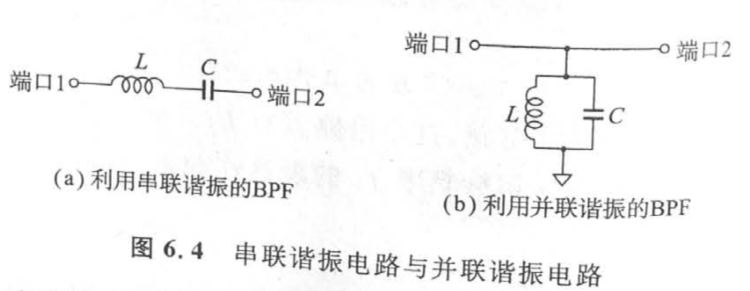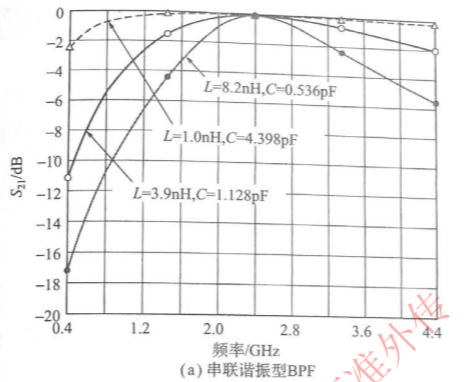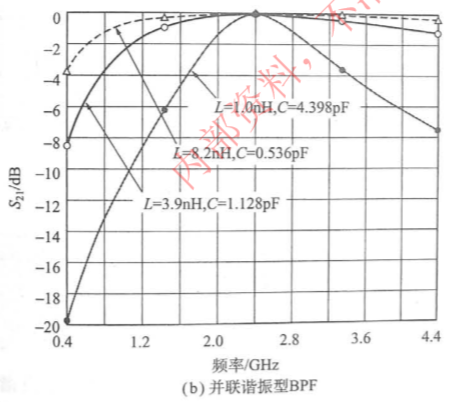串联谐振与并联谐振的组合电路：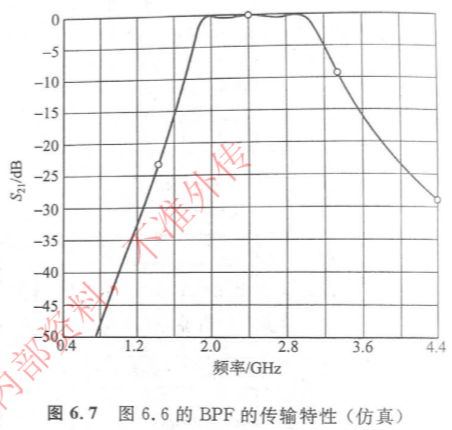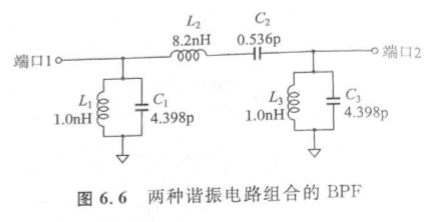五、介质滤波器：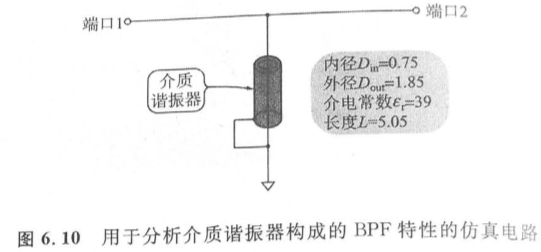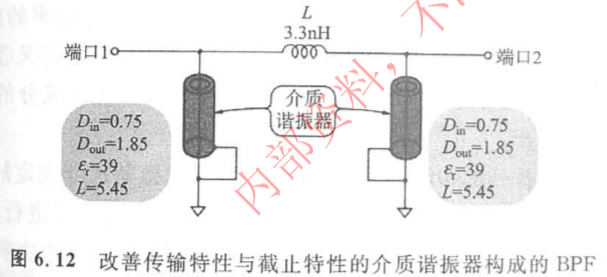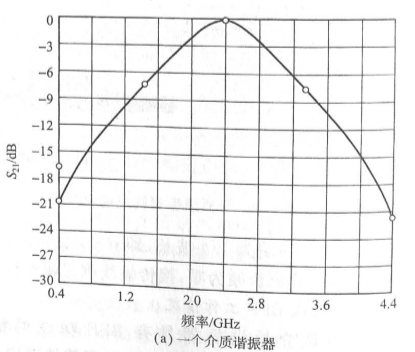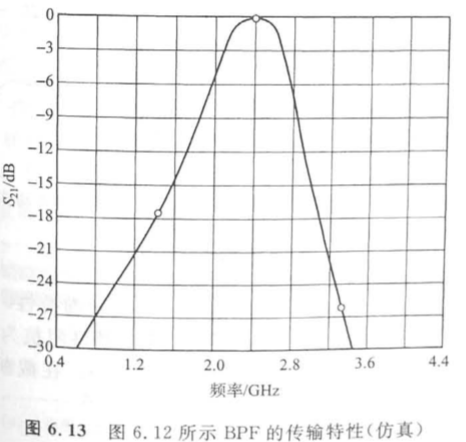六、微带线制作滤波器：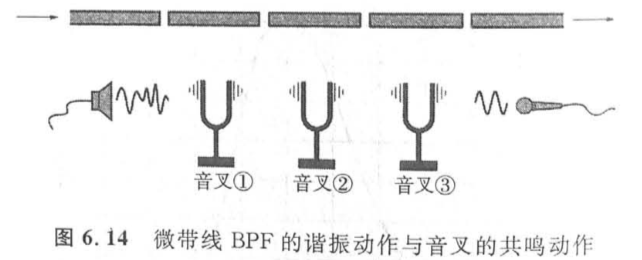展开全文微带线滤波器 介质滤波器 谐振电路
• 低通滤波器 允许低于截止频率的信号通过，但高于截止频率的信号不能通过的电子滤波装置。...带通滤波器 允许特定频率的信号通过。是指能通过某一频率范围内的频率分量、但将其他范围的频率分...


低通滤波器

允许低于截止频率的信号通过，但高于截止频率的信号不能通过的电子滤波装置。

高通滤波器

允许高于截止频率的信号通过。是一种让某一频率以上的信号分量通过，而对该频率以下的信号分量大大抑制的电容、电感与电阻等器件的组合装置。其特性在时域及频域中可分别用冲激响应及频率响应描述。

带通滤波器

允许特定频率的信号通过。是指能通过某一频率范围内的频率分量、但将其他范围的频率分量衰减到极低水平的滤波器，与带阻滤波器的概念相对。一个模拟带通滤波器的例子是电阻-电感-电容电路(RLC circuit)。这些滤波器也可以用低通滤波器同高通滤波器组合来产生。


展开全文• 带通滤波器电路图设计（一） 传统的带通滤波器设计方法中涉及了很多复杂的理论分析和计算。针对上述缺点，介绍一种使用EDA软件进行带通滤波器的设计方案，详细阐述了使用FilterPro软件进行有源带通滤波器电路的设计...
带通滤波器电路图设计（一）

传统的带通滤波器设计方法中涉及了很多复杂的理论分析和计算。针对上述缺点，介绍一种使用EDA软件进行带通滤波器的设计方案，详细阐述了使用FilterPro软件进行有源带通滤波器电路的设计步骤，然后给出了在Proteus中对所设计的滤波器进行仿真分析和测试的方法。测试结果表明，使用该方法设计的带通滤波器具有性能稳定。设计难度小等优点，也为滤波器的设计提供了一个新的思路。

带通滤波器是一种仅允许特定频率通过，同时对其余频率的信号进行有效抑制的电路。由于它对信号具有选择性，故而被广泛地应用现在电子设计中。但是，带通滤波器的种类繁多，各个类型的设计差异也很大，这就导致了在传统滤波器的设计方法中不可避免地要进行大量的理论计算与分析，不但损失了宝贵的时间，同时也提升了电路的设计门槛。为了解决上述弊端，本文介绍了一种使用FilterPro和Proteus相结合的有源带通滤波器的设计方案，随着EDA技术的不断发展，这种方法的优势也将越来越明显。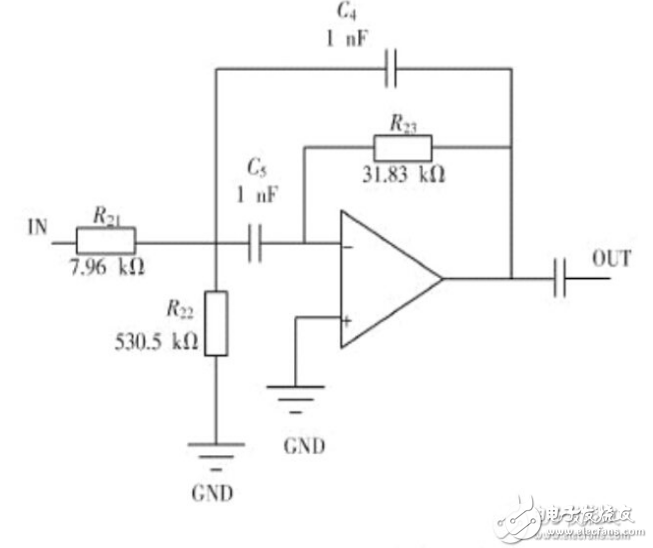图1  使用理想运放的带通滤波器

电路原理图如图1所示。然后可在Proteus中搭建电路进行仿真分析，前面已经提到，FilterPro生成的滤波器中的运放使用的理想运放模型，所以仿真时需要先用理想运放进行分析，然后再进行替换。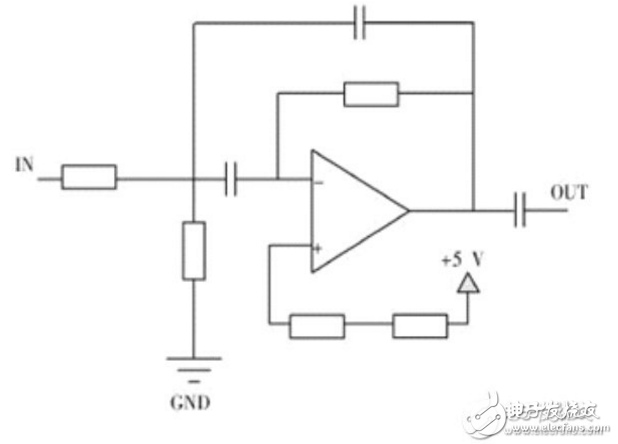图2  实际搭建的滤波器电路

设计中运放选择TI产品典型的通用双放LM358，LM358里面包括两个高增益、独立的、内部频率补偿的双运放，适用于电压范围很宽的单电源，而且也适用于双电源工作方式，特点方面具有低输入偏置电流、低输入失调电压和失调电流，它的共模输入电压范围较宽，差模输入电压范围等于电源电压范围，单电源供电电压3-32V，双电源供电±1.5-±16V，单位增益带宽为1MHz，适用于一般的带通滤波器的设计，同时具有低功耗的功能，对于设计阶数相对高一些的带通滤波器的话，可以选用TI的四运放LM324，其性能与LM358大体相同，应用起来节省空间。对于运放的要求此设计不是特别高，只要运放的频率满足低通的截止频率即可，如果精确度要求高的话那么首先运放的供电电压要足够稳定，或者选择精密运放，如TLC274A，否则通用的即可，例如推荐TI的LM224四运放。

巴特沃斯带通滤波器幅频响应在通带中具有最平幅度特性，但是从通带到阻带衰减较慢，如果对于过渡带要求稍高，可以增加阶数来实现，否则改选用切比雪夫滤波电路。

下面讨论设计两种带通滤波器，其一为二阶低通滤波器和二阶高通滤波器组成的四阶带通滤波器，如下图：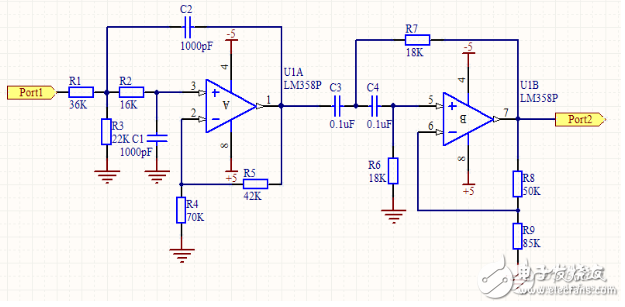图 3  四阶带通滤波器

参数选择与计算：

对于低通滤波器的设计，电容一般选取1000pF，对于高通滤波器的设计，电容一般选取0.1uF，然后根据公式R=1/2Πfc计算得出与电容相组合的电阻值，即得到此图中R2、R6和R7，为了消除运放的失调电流造成的误差，尽量是运放同相输入端与反向输入端对地的直流电阻基本相等，同时巴特沃斯滤波器阶数与增益有一定的关系（见表1），根据这两个条件可以列出两个等式：30=R4*R5/（R4+R5），R5=R4（A-1），36=R8*R9/（R8+R9），R8=R9（A-1）由此可以解出R4、R5、R8、R9，原则是根据现实情况稍调整电阻值保持在一定限度内即可，不要相差太大，注意频率不要超过运放的标定频率。

表1巴特沃斯低通、高通电路阶数与增益的关系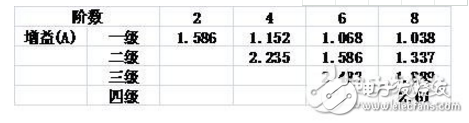其二是二阶有源带通滤波器，只用一个放大区间，如下图：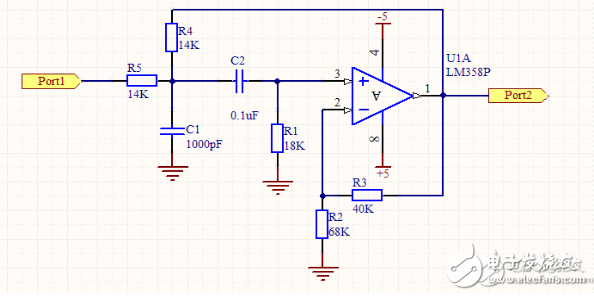图4  二阶带通滤波器

带通滤波器电路图设计（二）

由图（1）所示带通滤波电路的幅频响应与高通、低通滤波电路的幅频响应进行比较，不难发现低通与高通滤波电路相串联如图（2），可以构成带通滤波电路，条件是低通滤波电路的截止角频率WH大于高通电路的截止角频率WL，两者覆盖的通带就提供了一个带通响应。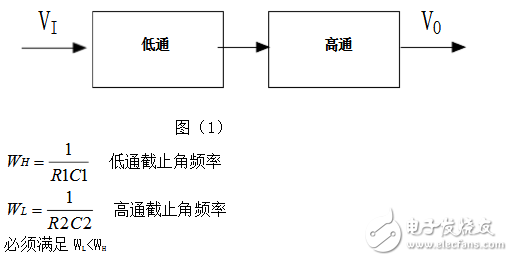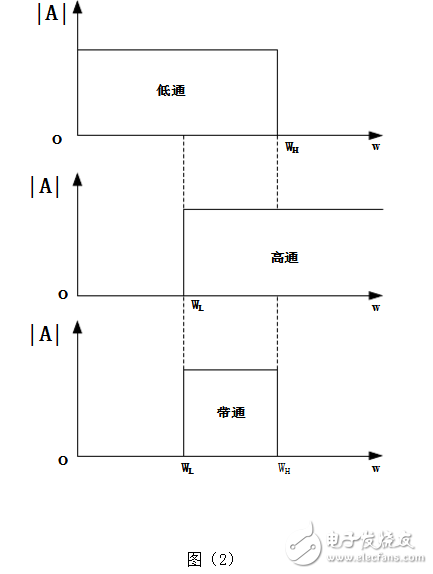这是一个通带频率范围为100HZ-10KHZ的带通滤波电路，在通带内我们设计为单位增益。根据题意，在频率低端f=10HZ时，幅频响应至少衰减26dB。在频率高端f=100KHZ时，幅频响应要求衰减不小于16dB。因此可以选择一个二阶高通滤波电路的截止频率fH=10KHZ，一个二阶低通滤波电路的fL=100HZ，有源器件仍选择运放LF142，将这两个滤波电路串联如图所示，就构成了所要求的带通滤波电路。

由巴特沃斯低通、高通电路阶数n与增益的关系知Avf1=1.586，因此，由两级串联的带通滤波电路的通带电压增益（Avf1）2=（1.586）2=2.515，由于所需要的通带增益为0dB，因此在低通滤波器输入部分加了一个由电阻R1、R2组成的分压器。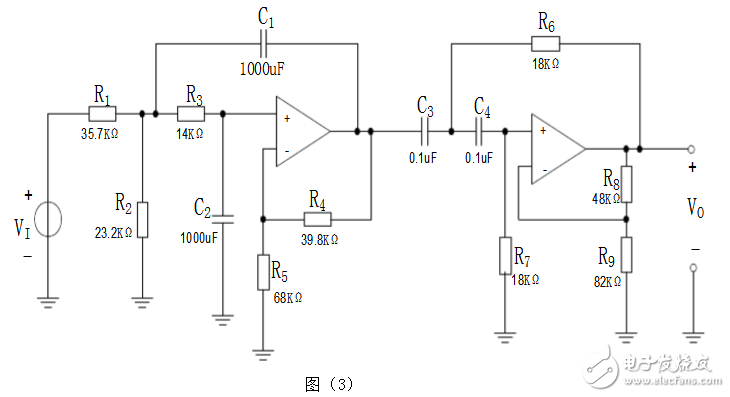元件参数的选择和计算

在选用元件时，应当考虑元件参数误差对传递函数带来的影响。现规定选择电阻值的容差为1%，电容值的容差为5%。由于每一电路包含若干电阻器和两个电容器，预计实际截止频率可能存在较大的误差（也许是+10%）。为确保在100Hz和10kHz处的衰减不大于3dB.现以额定截止频率90Hz和1kHz进行设计。

前已指出，在运放电路中的电阻不宜选择过大或较小。一般为几千欧至几十千欧较合适。因此，选择低通级电路的电容值为1000pF，高通级电路的电容值为0.1μF，然后由式RCWC1可计算出精确的电阻值。

对于低通级由于已知c=1000pF和fh=11kHz，由式RCWC1算得R3=14.47kΩ，先选择标准电阻值R3=14.0kΩ。对于高通级可做同样的计算。由于已知C=0.1μF和fL=90Hz，可求出R7=R8≈18kΩ。

考虑到已知Avf1=1.586，同时尽量要使运放同相输入端和反相输入端对地的直流电阻基本相等，现选择R5=68k，R10=82k，由此可算出R4=（Avf1-1）R5≈39.8k，R9=（Avf1-1）R10≈48k，其容差为1％。

设计完成的电路如图所示。信号源vI通过R1和R2进行衰减，它的戴维宁电阻是R1和R2的并联值，这个电阻应当等于低通级电阻R3（=14k）。因此，有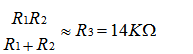由于整个滤波电路通带增益是电压分压器比值和滤波器部分增益的乘积，且应等于单位增益，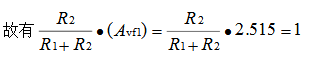联解式和，并选择容差为1％的额定电阻值，得R1=35.7kΩ和R2=23.2kΩ。

带通滤波器电路图设计（三）

实用的带通滤波器电路原理图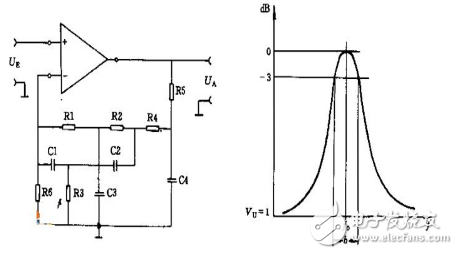该电路在负反馈支路上是一个带阻滤波齐器，以使其只允许通过被反馈支路阻断的频率信号。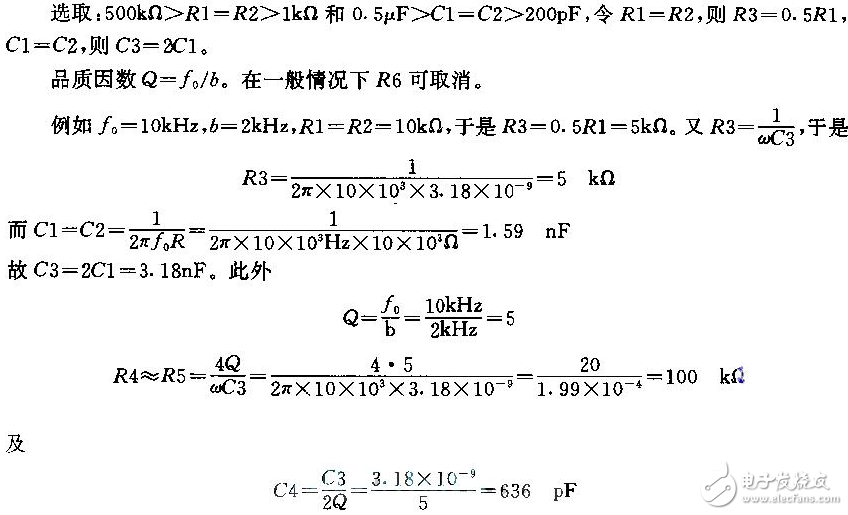展开全文• 下两个滤波器都是切比雪夫I型数字滤波器，不是巴特沃尔滤波器，请使用者注意！1.低通滤波器使用说明：将下列代码幅值然后以m文件保存，文件名要与函数名相同，这里函数名：lowp。function y=lowp(x,f1,f3,rp,rs,Fs) ...
本文为转载内容，原文地址为点击打开链接。下两个滤波器都是切比雪夫I型数字滤波器，不是巴特沃尔滤波器，请使用者注意！1.低通滤波器使用说明：将下列代码幅值然后以m文件保存，文件名要与函数名相同，这里函数名：lowp。function y=lowp(x,f1,f3,rp,rs,Fs)
%低通滤波
%使用注意事项：通带或阻带的截止频率的选取范围是不能超过采样率的一半
%即，f1,f3的值都要小于 Fs/2
%x:需要带通滤波的序列
% f 1：通带截止频率
% f 3：阻带截止频率
%rp：边带区衰减DB数设置
%rs：截止区衰减DB数设置
%FS：序列x的采样频率
% rp=0.1;rs=30;%通带边衰减DB值和阻带边衰减DB值
% Fs=2000;%采样率
%
wp=2*pi*f1/Fs;
ws=2*pi*f3/Fs;
% 设计切比雪夫滤波器；
[n,wn]=cheb1ord(wp/pi,ws/pi,rp,rs);
[bz1,az1]=cheby1(n,rp,wp/pi);
%查看设计滤波器的曲线
[h,w]=freqz(bz1,az1,256,Fs);
h=20*log10(abs(h));
figure;plot(w,h);title('所设计滤波器的通带曲线');grid on;
%
y=filter(bz1,az1,x);%对序列x滤波后得到的序列y
end--------------------------------------低通滤波器使用例子的代码fs=2000;
t=(1:fs)/fs;
ff1=100;
ff2=400;
x=sin(2*pi*ff1*t)+sin(2*pi*ff2*t);
figure;
subplot(211);plot(t,x);
subplot(212);hua_fft(x,fs,1);
%低通测试
% y=filter(bz1,az1,x);
y=lowp(x,300,350,0.1,20,fs);
figure;
subplot(211);plot(t,y);
subplot(212);hua_fft(y,fs,1);%hua_fft()函数是画频谱图的函数，代码在下面给出，要保存为m文件调用%这段例子还调用了我自己写的专门画频谱图的函数，也给出，不然得不出我的结果;%画信号的幅频谱和功率谱
%频谱使用matlab例子表示
function hua_fft(y,fs,style,varargin)
%当style=1,画幅值谱；当style=2,画功率谱;当style=其他的，那么花幅值谱和功率谱
%当style=1时，还可以多输入2个可选参数
%可选输入参数是用来控制需要查看的频率段的
%第一个是需要查看的频率段起点
%第二个是需要查看的频率段的终点
%其他style不具备可选输入参数，如果输入发生位置错误
nfft=2^nextpow2(length(y));%找出大于y的个数的最大的2的指数值（自动进算最佳FFT步长nfft）
%nfft=1024;%人为设置FFT的步长nfft
y=y-mean(y);%去除直流分量
y_ft=fft(y,nfft);%对y信号进行DFT，得到频率的幅值分布
y_p=y_ft.*conj(y_ft)/nfft;%conj()函数是求y函数的共轭复数，实数的共轭复数是他本身。
y_f=fs*(0:nfft/2-1)/nfft;�T变换后对应的频率的序列
% y_p=y_ft.*conj(y_ft)/nfft;%conj()函数是求y函数的共轭复数，实数的共轭复数是他本身。
if style==1
ifnargin==3
plot(y_f,2*abs(y_ft(1:nfft/2))/length(y));%matlab的帮助里画FFT的方法
%ylabel('幅值');xlabel('频率');title('信号幅值谱');
%plot(y_f,abs(y_ft(1:nfft/2)));%论坛上画FFT的方法
else
f1=varargin{1};
fn=varargin{2};
ni=round(f1 * nfft/fs+1);
na=round(fn * nfft/fs+1);
plot(y_f(ni:na),abs(y_ft(ni:na)*2/nfft));
end

elseif style==2
plot(y_f,y_p(1:nfft/2));
%ylabel('功率谱密度');xlabel('频率');title('信号功率谱');
else
subplot(211);plot(y_f,2*abs(y_ft(1:nfft/2))/length(y));
ylabel('幅值');xlabel('频率');title('信号幅值谱');
subplot(212);plot(y_f,y_p(1:nfft/2));
ylabel('功率谱密度');xlabel('频率');title('信号功率谱');
end
end下面三幅图分别是滤波前的时频图，滤波器的滤波特性曲线图和滤波后的时频图，通过图可以看出成功留下了100Hz的低频成分而把不要的高频成分去除了。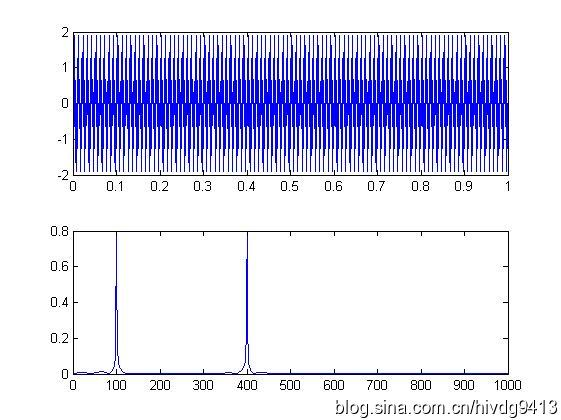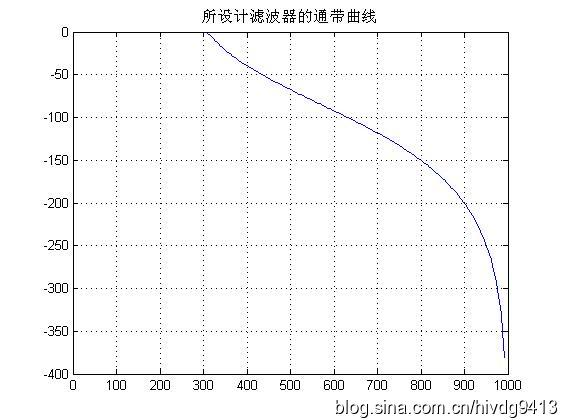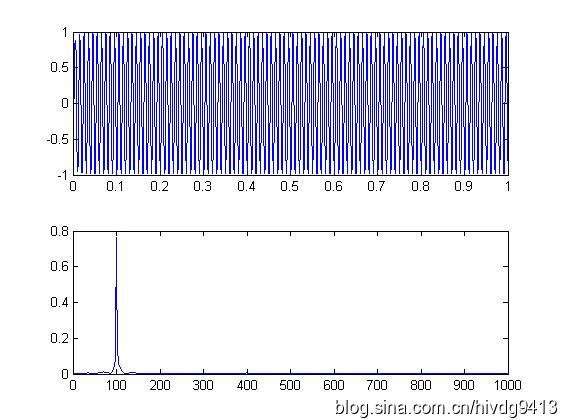2.高通滤波器function y=highp(x,f1,f3,rp,rs,Fs)
%高通滤波
%使用注意事项：通带或阻带的截止频率的选取范围是不能超过采样率的一半
%即，f1,f3的值都要小于 Fs/2
%x:需要带通滤波的序列
% f 1：通带截止频率
% f 2：阻带截止频率
%rp：边带区衰减DB数设置
%rs：截止区衰减DB数设置
%FS：序列x的采样频率
% rp=0.1;rs=30;%通带边衰减DB值和阻带边衰减DB值
% Fs=2000;%采样率
%
wp=2*pi*f1/Fs;
ws=2*pi*f3/Fs;
% 设计切比雪夫滤波器；
[n,wn]=cheb1ord(wp/pi,ws/pi,rp,rs);
[bz1,az1]=cheby1(n,rp,wp/pi,'high');

%查看设计滤波器的曲线
[h,w]=freqz(bz1,az1,256,Fs);
h=20*log10(abs(h));
figure;plot(w,h);title('所设计滤波器的通带曲线');grid on;
y=filter(bz1,az1,x);
end下面是高通滤波器的例子fs=2000;
t=(1:fs)/fs;
ff1=100;
ff2=400;
x=sin(2*pi*ff1*t)+sin(2*pi*ff2*t);
figure;
subplot(211);plot(t,x);
subplot(212);hua_fft(x,fs,1);

%------高通测试
z=highp(x,350,300,0.1,20,fs);
figure;
subplot(211);plot(t,z);
subplot(212);hua_fft(z,fs,1);下面三幅图分别是滤波前的时频图，滤波器的滤波特性曲线图和滤波后的时频图，通过图可以看出成功留下了400Hz的高频成分而把不要的低频成分100Hz去除了。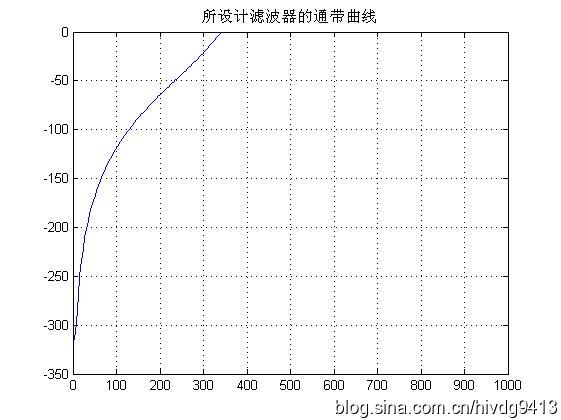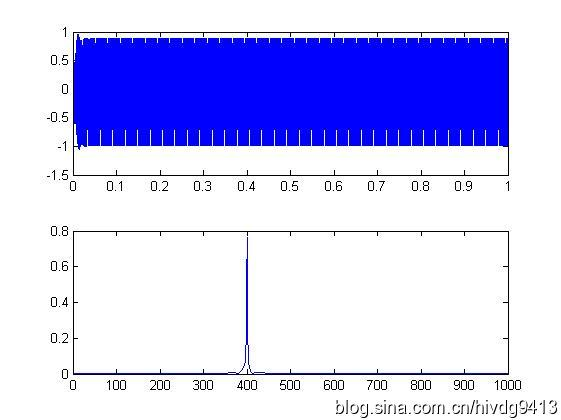3.带通滤波器function y=bandp(x,f1,f3,fsl,fsh,rp,rs,Fs)
%带通滤波
%使用注意事项：通带或阻带的截止频率与采样率的选取范围是不能超过采样率的一半
%即，f1,f3,fs1,fsh,的值小于 Fs/2
%x:需要带通滤波的序列
% f 1：通带左边界
% f 3：通带右边界
% fs1：衰减截止左边界
% fsh：衰变截止右边界
%rp：边带区衰减DB数设置
%rs：截止区衰减DB数设置
%FS：序列x的采样频率
% f1=300;f3=500;%通带截止频率上下限
% fsl=200;fsh=600;%阻带截止频率上下限
% rp=0.1;rs=30;%通带边衰减DB值和阻带边衰减DB值
% Fs=2000;%采样率
%
wp1=2*pi*f1/Fs;
wp3=2*pi*f3/Fs;
wsl=2*pi*fsl/Fs;
wsh=2*pi*fsh/Fs;
wp=[wp1 wp3];
ws=[wsl wsh];
%
% 设计切比雪夫滤波器；
[n,wn]=cheb1ord(ws/pi,wp/pi,rp,rs);
[bz1,az1]=cheby1(n,rp,wp/pi);
%查看设计滤波器的曲线
[h,w]=freqz(bz1,az1,256,Fs);
h=20*log10(abs(h));
figure;plot(w,h);title('所设计滤波器的通带曲线');grid on;
y=filter(bz1,az1,x);
end带通滤波器使用例子%--------------
%带通滤波器测试程序
fs=2000;
t=(1:fs)/fs;
ff1=100;
ff2=400;
ff3=700;
x=sin(2*pi*ff1*t)+sin(2*pi*ff2*t)+sin(2*pi*ff3*t);
figure;
subplot(211);plot(t,x);
subplot(212);hua_fft(x,fs,1);
% y=filter(bz1,az1,x);
y=bandp(x,300,500,200,600,0.1,30,fs);
figure;
subplot(211);plot(t,y);
subplot(212);hua_fft(y,fs,1);%调用到的hua_fft()函数代码如下function hua_fft(y,fs,style,varargin)
%当style=1,画幅值谱；当style=2,画功率谱;当style=其他的，那么花幅值谱和功率谱
%当style=1时，还可以多输入2个可选参数
%可选输入参数是用来控制需要查看的频率段的
%第一个是需要查看的频率段起点
%第二个是需要查看的频率段的终点
%其他style不具备可选输入参数，如果输入发生位置错误
nfft=2^nextpow2(length(y));%找出大于y的个数的最大的2的指数值（自动进算最佳FFT步长nfft）
%nfft=1024;%人为设置FFT的步长nfft
y=y-mean(y);%去除直流分量
y_ft=fft(y,nfft);%对y信号进行DFT，得到频率的幅值分布
y_p=y_ft.*conj(y_ft)/nfft;%conj()函数是求y函数的共轭复数，实数的共轭复数是他本身。
y_f=fs*(0:nfft/2-1)/nfft;�T变换后对应的频率的序列
% y_p=y_ft.*conj(y_ft)/nfft;%conj()函数是求y函数的共轭复数，实数的共轭复数是他本身。
if style==1
ifnargin==3
plot(y_f,2*abs(y_ft(1:nfft/2))/length(y));%matlab的帮助里画FFT的方法
%ylabel('幅值');xlabel('频率');title('信号幅值谱');
%plot(y_f,abs(y_ft(1:nfft/2)));%论坛上画FFT的方法
else
f1=varargin{1};
fn=varargin{2};
ni=round(f1 * nfft/fs+1);
na=round(fn * nfft/fs+1);
plot(y_f(ni:na),abs(y_ft(ni:na)*2/nfft));
end

elseif style==2
plot(y_f,y_p(1:nfft/2));
%ylabel('功率谱密度');xlabel('频率');title('信号功率谱');
else
subplot(211);plot(y_f,2*abs(y_ft(1:nfft/2))/length(y));
ylabel('幅值');xlabel('频率');title('信号幅值谱');
subplot(212);plot(y_f,y_p(1:nfft/2));
ylabel('功率谱密度');xlabel('频率');title('信号功率谱');
end
end运行结果如下图，第一幅是滤波前测试信号的时频图，第二幅是滤波器的滤波曲线图，第三幅是经滤波后的测试信号时频图。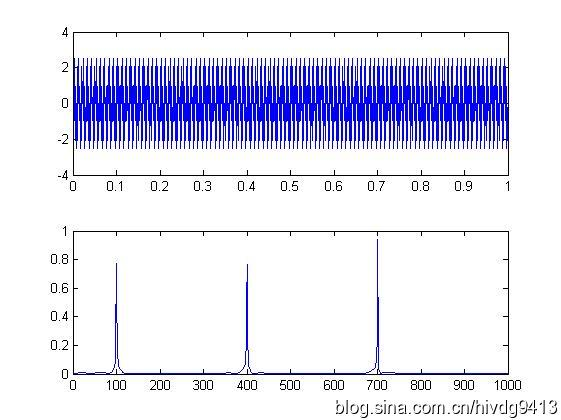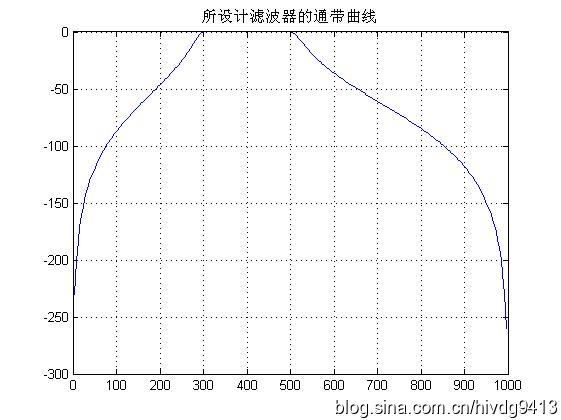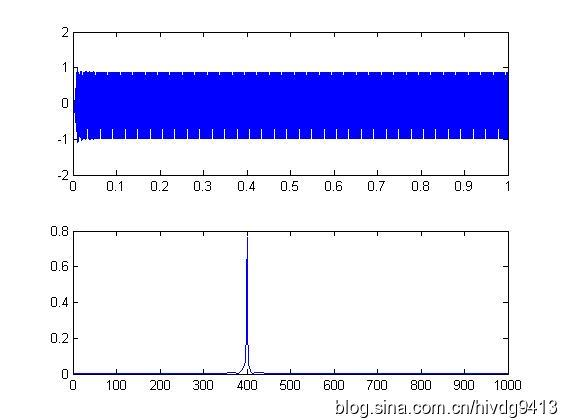4.带阻滤波器function y=bands(x,f1,f3,fsl,fsh,rp,rs,Fs)
%带阻滤波
%使用注意事项：通带或阻带的截止频率与采样率的选取范围是不能超过采样率的一半
%即，f1,f3,fs1,fsh,的值小于 Fs/2
%x:需要带通滤波的序列
% f 1：通带左边界
% f 3：通带右边界
% fs1：衰减截止左边界
% fsh：衰变截止右边界
%rp：边带区衰减DB数设置
%rs：截止区衰减DB数设置
%FS：序列x的采样频率
% f1=300;f3=500;%通带截止频率上下限
% fsl=200;fsh=600;%阻带截止频率上下限
% rp=0.1;rs=30;%通带边衰减DB值和阻带边衰减DB值
% Fs=2000;%采样率
%
wp1=2*pi*f1/Fs;
wp3=2*pi*f3/Fs;
wsl=2*pi*fsl/Fs;
wsh=2*pi*fsh/Fs;
wp=[wp1 wp3];
ws=[wsl wsh];
%
% 设计切比雪夫滤波器；
[n,wn]=cheb1ord(ws/pi,wp/pi,rp,rs);
[bz1,az1]=cheby1(n,rp,wp/pi,'stop');
%查看设计滤波器的曲线
[h,w]=freqz(bz1,az1,256,Fs);
h=20*log10(abs(h));
figure;plot(w,h);title('所设计滤波器的通带曲线');grid on;
y=filter(bz1,az1,x);
end使用例子%带阻滤波器测试
fs=1000;
t=(1:fs)/fs;
y=sin(2*pi*100*t)+sin(2*pi*150*t)+sin(2*pi*200*t);
figure;hua_fft(y,fs,1);
z=bands(y,110,190,140,160,0.1,30,fs);
figure;hua_fft(z,fs,1);运行结果如下图，第一幅是滤波前测试信号的频谱图，第二幅是滤波器的滤波曲线图，第三幅是经滤波后的测试信号频谱图。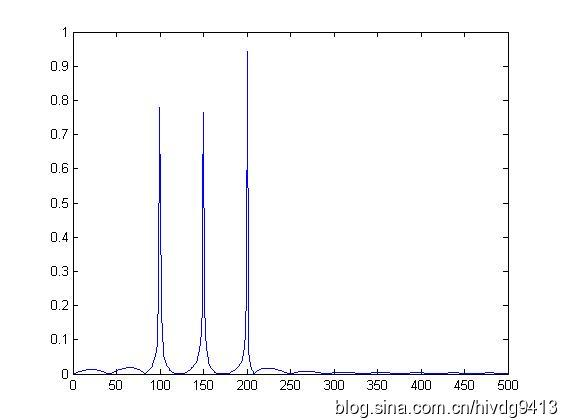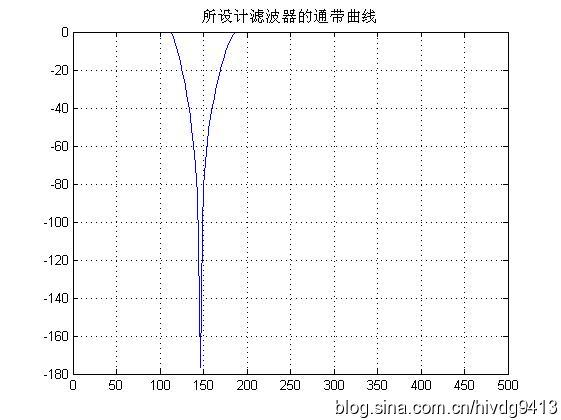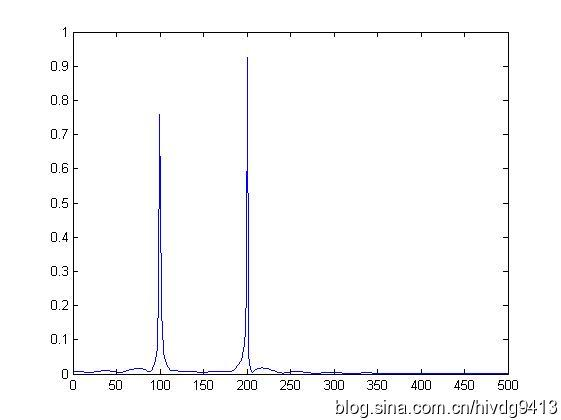展开全文低通滤波器 高通滤波器 带阻滤波器 matlab
• 基于MATLAB对带限信号进行滤波处理并作出时域图和频域的图像，对信号进行处理并分析和对比。
• 应用切比雪夫滤波器，设计了带通滤波器 function y=bandp(x,f1,f3,fsl,fsh,rp,rs,Fs) %带通滤波 %使用注意事项：通带或阻带的截止频率与采样率的选取范围是不能超过采样率的一半 %即，f1,f3,fs1,fsh,的值小于 Fs/2 %...
• 随机信号通过带通滤波器 题目描述： 信号为两个正弦信号之和，一个振幅为1，频率为60Hz，另一个振幅为0.5，频率为40Hz；加性噪声是均值为0，方差为4的高斯白噪声，一起调制到9.5kHz的载波上，经过带宽为200Hz、中心...
• 自己编写的小程序，matlab程序，带通滤波器，可设置最小截止频率和最大截止频率。 ps。不附带信号文件，很简单的原理，旨在指导带通滤波器的编程思路与原理
• ## FIR 带通滤波器设计

万次阅读 2018-09-05 17:29:50
%本文将针对一个含有 5Hz 、 15Hz 和 30Hz 的混和正弦波信号， 设计一个 FIR 带通滤波器， %给出利用 MATLAB 实现的三种方法： 程序设计法、 FDATool 设计法和 SPTool 设计法。 参 %数要求：采样频率 fs=100Hz ，...
• ## 二阶有源带通滤波器滤波原理

万次阅读 多人点赞 2017-01-18 17:20:35
请注意有源滤波器因为运放的有效识别电压和响应频率的影响，适用于低频信号的滤波，对于高频信号最好使用无缘滤波。 名词解释 一阶低通滤波器，包含一组RC构成的滤波器，将谐波过滤一次；两阶低通滤波器，包含两组...
• 滤波器在电子线路中用得特别多，通信电路更是多如牛毛。这是一个关于二阶有源带通滤波器设计及参数计算的资料。希望对大家有用。
• 简单记录下在matlab上如何设计出模拟的带通滤波器，包括：巴特沃斯滤波器、切比雪夫I型滤波器、切比雪夫II型滤波器、椭圆型滤波器。 代码如下： %设计带通滤波器 %巴特沃斯、切比雪夫I型、切比雪夫II型、椭圆...
• 设计了一个f0=40K的带通滤波器 同相输入压控电压源二阶有源二阶带通滤波器 调信号发生器频率 示波器双踪比较看 中心频率怎么都不对 在35K左右 于是改用matlab仿真 结果为40K 改用频响分析 数据输出： "FREQ","Vout" ...
• 1.1 Ⅰ、Ⅱ型理想带通滤波器的单位冲激响应 计算 1 1.2 Ⅲ、Ⅳ型理想带通滤波器的单位冲激响应hd(n)计算 2 1.3 滤波器的幅值响应 2 2 . fir带通滤波器的窗函数设计 3 2.1利用三角窗设计Ⅲ型数字带通滤波器 4 2.2利用...
• 设计带通滤波器，MATLAB程序.这个程序简单明了，能够很容易的进行滤波。
• 看到一个带通滤波器的设计如下，开始研究是如何设计的 代码： band_low = 0.3; band_high = 1.2; fs_origin = 25; hd_band = design(fdesign.bandpass('N,F3dB1,F3dB2',4,band_low,band_high,fs_origin),'...
• 二阶巴特沃斯带通滤波器仿真电路图，二阶巴特沃斯带通滤波器仿真电路图
• FIR带通滤波器的M文件源代码，系数通过MATLAB的工具箱设计得到。
• Butterworth巴特沃斯带通滤波器音频分离Matlab程序，FFT频域处理，音频分离。
• 1、熟悉Multisim9软件的使用方法。 2、熟悉二阶低通滤波器的特性 3、掌握二阶低通滤波器的幅频特性。...